## Brocard PointsThe first Brocard point is the interior point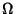(oror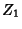) of a Triangle for which the Angles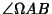,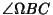, and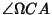are equal. The second Brocard point is the interior point(oror) for which the Angles,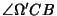, and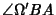are equal. The Angles in both cases are equal to the Brocard Angle,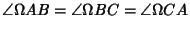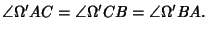The first two Brocard points are Isogonal Conjugates (Johnson 1929, p. 266).Letbe the Circle which passes through the verticesandand is Tangent to the lineat, and similarly forand. Then the Circles,, and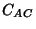intersect in the first Brocard point. Similarly, letbe the Circle which passes through the verticesandand is Tangent to the lineat, and similarly forand. Then the Circles,, andintersect in the second Brocard points(Johnson 1929, pp. 264-265).The Pedal Triangles ofandare congruent, and Similar to the Triangle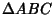(Johnson 1929, p. 269). Lengths involving the Brocard points include(1)(2)

Brocard's third point is related to a given Triangle by the Triangle Center Function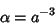(3)

(Casey 1893, Kimberling 1994). The third Brocard point(oror) is Collinear with the Spieker Center and the Isotomic Conjugate Point of its Triangle's Incenter.

References

Casey, J. A Treatise on the Analytical Geometry of the Point, Line, Circle, and Conic Sections, Containing an Account of Its Most Recent Extensions, with Numerous Examples, 2nd ed., rev. enl. Dublin: Hodges, Figgis, & Co., p. 66, 1893.

Johnson, R. A. Modern Geometry: An Elementary Treatise on the Geometry of the Triangle and the Circle. Boston, MA: Houghton Mifflin, pp. 263-286, 1929.

Kimberling, C. Central Points and Central Lines in the Plane of a Triangle.'' Math. Mag. 67, 163-187, 1994.

Stroeker, R. J. Brocard Points, Circulant Matrices, and Descartes' Folium.'' Math. Mag. 61, 172-187, 1988.